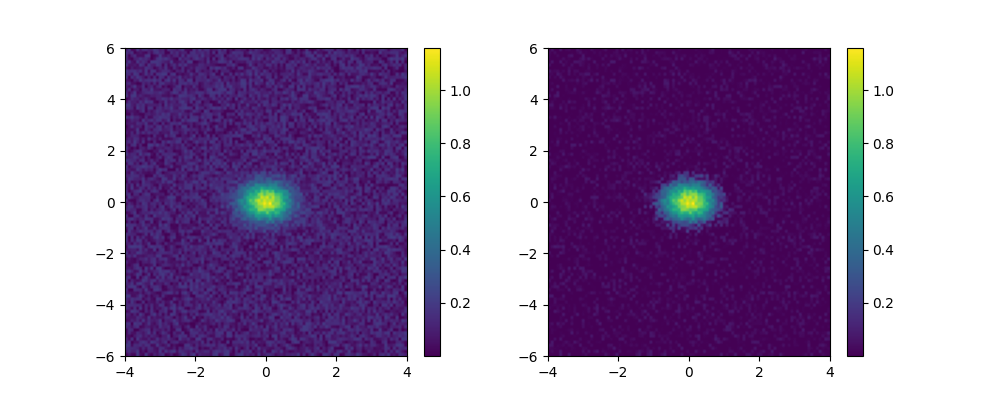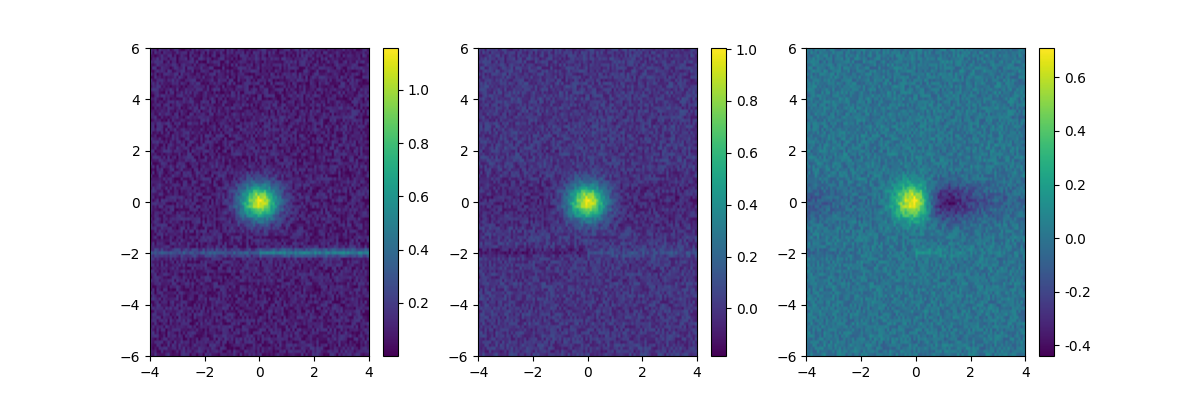# Filter¶

‘audio.utils.filter’ module within the ketos library

This module provides utilities for manipulating and filtering waveforms and spectrograms.

ketos.audio.utils.filter.apply_median_filter(img, row_factor=3, col_factor=4)[source]

Discard pixels that are lower than the median threshold.

The resulting image will have 0s for pixels below the threshold and 1s for the pixels above the threshold.

Note: Code adapted from Kahl et al. (2017)
Args:
imgnumpy array

Array containing the img to be filtered. OBS: Note that contents of img are modified by call to function.

row_factor: int or float

Factor by which the row-wise median pixel value will be multiplied in orther to define the threshold.

col_factor: int or float

Factor by which the col-wise median pixel value will be multiplied in orther to define the threshold.

Returns:
filtered_img: numpy array

The filtered image with 0s and 1s.

Example:
>>> from ketos.audio.utils.filter import apply_median_filter
>>> img = np.array([[1,4,5],
...                 [3,5,1],
...                 [1,0,9]])
>>> img_fil = apply_median_filter(img, row_factor=1, col_factor=1)
>>> print(img_fil)
[[0 0 0]
[0 1 0]
[0 0 1]]

ketos.audio.utils.filter.apply_preemphasis(sig, coeff=0.97)[source]

Apply pre-emphasis to signal

Args:
signumpy array

1-d array containing the signal.

coeff: float

The preemphasis coefficient. If set to 0, no preemphasis is applied (the output will be the same as the input).

Returns:
emphasized_signalnumpy array

The filtered signal.

Example:

>>> from ketos.audio.utils.filter import apply_preemphasis
>>> sig = np.array([1,2,3,4,5])
>>> sig_new = apply_preemphasis(sig, coeff=0.95)
>>> print(sig_new)
[1.   1.05 1.1  1.15 1.2 ]

ketos.audio.utils.filter.blur_image(img, size=20, sigma=5, gaussian=True)[source]

Smooth the input image using a median or Gaussian blur filter.

Note that the input image is recasted as np.float32.

This is essentially a wrapper around the scipy.ndimage.median_filter and scipy.ndimage.gaussian_filter methods.

For further details, see https://docs.scipy.org/doc/scipy/reference/ndimage.html

Args:
imgnumpy array

Image to be processed.

size: int

Only used by the median filter. Describes the shape that is taken from the input array, at every element position, to define the input to the filter function.

sigma: float or array

Only used by the Gaussian filter. Standard deviation for Gaussian kernel. May be given as a single number, in which case all axes have the same standard deviation, or as an array, allowing for the axes to have different standard deviations.

Gaussian: bool

Switch between median and Gaussian (default) filter

Returns:
blur_img: numpy array

Blurred image.

Example:
>>> from ketos.audio.utils.filter import blur_image
>>> img = np.array([[0,0,0],
...                 [0,1,0],
...                 [0,0,0]])
>>> # blur using Gaussian filter with sigma of 0.5
>>> img_blur = blur_image(img, sigma=0.5)
>>> img_blur = np.around(img_blur, decimals=2) # only keep up to two decimals
>>> print(img_blur)
[[0.01 0.08 0.01]
[0.08 0.62 0.08]
[0.01 0.08 0.01]]

ketos.audio.utils.filter.enhance_signal(img, enhancement=1.0)[source]

Enhance the contrast between regions of high and low intensity, while preserving the range of pixel values.

Multiplies each pixel value by the factor,whereis the pixel value,is the pixel value median of the image, and, whereis the pixel value standard deviation of the image andis the enhancement parameter.

Some observations:

•is a smoothly increasing function from 0 to 1.

•, i.e. the mediandemarks the transition from “low intensity” to “high intensity”.

• The smaller the width,, the faster the transition from 0 to 1.

Args:
imgnumpy array

Image to be processed.

enhancement: float

Parameter determining the amount of enhancement.

Returns:
img_en: numpy array

Enhanced image.

Example:
>>> from ketos.audio.utils.filter import enhance_signal, plot_image
>>> #create an image
>>> x = np.linspace(-4,4,100)
>>> y = np.linspace(-6,6,100)
>>> x,y = np.meshgrid(x,y,indexing='ij')
>>> img = np.exp(-(x**2+y**2)/(2*0.5**2)) #symmetrical Gaussian
>>> img += 0.2 * np.random.rand(100,100)  #add some noise
>>> # apply enhancement
>>> img_enh = enhance_signal(img, enhancement=3.0)
>>> #draw the original image and its enhanced version
>>> import matplotlib.pyplot as plt
>>> fig, (ax1,ax2) = plt.subplots(1,2,figsize=(10,4)) #create canvas to draw on
>>> plot_image(img,fig,ax1,extent=(-4,4,-6,6))
>>> plot_image(img_enh,fig,ax2,extent=(-4,4,-6,6))
>>> fig.savefig("ketos/tests/assets/tmp/image_enhancement1.png")ketos.audio.utils.filter.filter_isolated_spots(img, struct=array([[1, 1, 1], [1, 1, 1], [1, 1, 1]]))[source]

Remove isolated spots from the image.

Args:
imgnumpy array

An array like object representing an image.

structnumpy array

A structuring pattern that defines feature connections. Must be symmetric.

Returns:
filtered_arraynumpy array

An array containing the input image without the isolated spots.

Example:
>>> from ketos.audio.utils.filter import filter_isolated_spots
>>> img = np.array([[0,0,1,1,0,0],
...                 [0,0,0,1,0,0],
...                 [0,1,0,0,0,0],
...                 [0,0,0,0,0,0],
...                 [0,0,0,1,0,0]])
>>> # remove pixels without neighbors
>>> img_fil = filter_isolated_spots(img)
>>> print(img_fil)
[[0 0 1 1 0 0]
[0 0 0 1 0 0]
[0 0 0 0 0 0]
[0 0 0 0 0 0]
[0 0 0 0 0 0]]

ketos.audio.utils.filter.plot_image(img, fig, ax, extent=None, xlabel='', ylabel='')[source]

Draw the image.

Args:
img: numpy array

Pixel values

fig: matplotlib.figure.Figure

Figure object

ax: matplotlib.axes.Axes

Axes object

extent: tuple(float,float,float,float)

Extent of axes, optional.

xlabel: str

Label for x axis, optional.

ylabel: str

Label for y axis, optional.

Returns:

None

ketos.audio.utils.filter.reduce_tonal_noise(img, method='MEDIAN', **kwargs)[source]

Reduce continuous tonal noise produced by e.g. ships and slowly varying background noise

Currently, offers the following two methods:

1. MEDIAN: Subtracts from each row the median value of that row.

2. RUNNING_MEAN: Subtracts from each row the running mean of that row.

The running mean is computed according to the formula given in Baumgartner & Mussoline, JASA 129, 2889 (2011); doi: 10.1121/1.3562166

Args:
img: numpy.array

Spectrogram image

method: str

Options are ‘MEDIAN’ and ‘RUNNING_MEAN’

Optional args:
time_const_len: int

Time constant in number of samples, used for the computation of the running mean. Must be provided if the method ‘RUNNING_MEAN’ is chosen.

Returns:
img_new: numpy array

Corrected spectrogram image

Example:
>>> import numpy as np
>>> from ketos.audio.utils.filter import reduce_tonal_noise, plot_image
>>> #create an image
>>> x = np.linspace(-4,4,100)
>>> y = np.linspace(-6,6,100)
>>> x,y = np.meshgrid(x,y,indexing='ij')
>>> img = np.exp(-(x**2+y**2)/(2*0.5**2)) #symmetrical Gaussian
>>> img += 0.2 * np.random.rand(100,100)  #add some flat noise
>>> #add tonal noise that exhibits sudden increase in amplitude
>>> img += 0.2 * (1 + np.heaviside(x,0.5)) * np.exp(-(y + 2.)**2/(2*0.1**2))
>>> #reduce tonal noise
>>> img_m = reduce_tonal_noise(img, method='MEDIAN')
>>> img_r = reduce_tonal_noise(img, method='RUNNING_MEAN', time_const_len=30)
>>> #draw the resulting images along with the original one
>>> import matplotlib.pyplot as plt
>>> fig, (ax1,ax2,ax3) = plt.subplots(1,3,figsize=(12,4)) #create canvas to draw on
>>> ext = (-4,4,-6,6)
>>> plot_image(img,fig,ax1,extent=ext)
>>> plot_image(img_m,fig,ax2,extent=ext)
>>> plot_image(img_r,fig,ax3,extent=ext)
>>> fig.savefig("ketos/tests/assets/tmp/image_tonal_noise_red1.png")ketos.audio.utils.filter.reduce_tonal_noise_running_mean(img, time_const_len)[source]

Reduce continuous tonal noise produced by e.g. ships and slowly varying background noise by subtracting from each row a running mean, computed according to the formula given in Baumgartner & Mussoline, Journal of the Acoustical Society of America 129, 2889 (2011); doi: 10.1121/1.3562166

Args:
img: numpy.array

Spectrogram image

time_const_len: int

Time constant in number of samples, used for the computation of the running mean. Must be provided if the method ‘RUNNING_MEAN’ is chosen.

Returns:
img_new2d numpy array

Corrected spetrogram image